﻿ Mind and InfinityPublications are Open
Access in this journal
Article Versions
Export Article
• Normal Style
• MLA Style
• APA Style
• Chicago Style
Research Article
Open Access Peer-reviewed

### Mind and Infinity

Abhijit Manohar
Applied Mathematics and Physics. 2021, 9(1), 16-27. DOI: 10.12691/amp-9-1-4
Received August 03, 2021; Revised September 05, 2021; Accepted September 15, 2021

### Abstract

The paper presents the general intuition of the concept of infinity. The Ramanujan’s Infinite series is reviewed in the light of proposed theory though the mathematical proof is presented more convincingly later in the article. The article discusses the cosmology and the size of universe and contrasts the finite vs. infinite universe arguments. The article questions and comments about the existence of transcendental numbers and Cantor’s Diagonal argument and also proves the problems with cardinality of infinity. It further gives other instantiations of intuitions and the consequences of mathematical infinity in limits, calculus, irrationals and series, respectively. The paper also suggests empirical methods for confirming the understanding of infinity presented in this paper in future. The 2 arguments – flexibility of mind (a priori intuition) and problem of the cardinality of infinity are presented and proved in this article. Both cognitive and mathematical explanation is provided to reinforce the idea that infinity is an a priori intuition without any physical counterpart.

### 1. Introduction and Theory

The infinite! No other question has ever moved so profoundly the spirit of man; no other idea has so fruitfully stimulated his intellect; yet no other concept stands in greater need of clarification than that of the infinite 1. We have tried to understand infinity since antiquity yet there is no consensus about it in the scientific community.

According to Anaximander of Miletus, the word apeiron stands for something unbounded, infinite, and indefinite or undefined 2. Apeiron characterized the primitive unity of all phenomena possessing no specific qualities. Pythagoreans went even further to ascribe pejorative connotations to the word infinite. Finite was good and infinite was evil. However, the physical world seemed to force upon them the concept of infinity by observations of infinite time, and boundless space. The non-observability of the end of universe attributes infinity to time. For a long time √2 was believed to be a rational number until it was found to be irrational after which it was not considered a number at all 3. Since then a lot of other irrational numbers have been found like the Golden ratio. In geometry, Aristotle accepted that points are on lines but points do not comprise the line and the continuous cannot be made of the discrete 2. In one of the 23 definitions in his exposition ‘Elements’, Euclid defined a point as that which has no part 4. This definition is based on the understanding of infinitesimal or infinite divisions of space. Galileo said, “It is wrong to speak of infinite quantities as being the one greater or less than or equal to the other.” 2. This statement by Galileo is important and will be discussed throughout this article. John Wallis first gave the symbol ∞ to infinity, representing a never-ending curve 2. Carl Gauss objected the use of infinity, “I protest against the use of an infinite quantity as an actual entity; this is never allowed in mathematics. The infinite is only a manner of speaking, in which one properly speaks of limits to which certain ratios can come as near as desired, while others are permitted to increase without bound.” The understanding of infinity has been enriched by many more mathematicians like Euler, Fermat etc.

The human reason evolved to match the external reality. Empiricism, as understood today, is a close picture of external reality than the a priori logic. It works better than logic (a priori understanding), much better than intuitive heuristics. The human mind has an uncanny ability and apparent conceivability to hold an “infinite object” in the default network also called the “imagination network” of the brain and reduce it to the smaller size and question what is outside the smaller size. The smaller size with its outside, which then is considered infinite as a whole, can be again reduced to the size of a same smaller size and again question what is outside the smaller size! There is always something outside anything shrunk to the smaller size in the mind. There is an infinite regress to this process. In the same way, the converse, that human mind can hold a finite object in the mind and then expand it to an infinite size within the mind is true.

• Figure 1. Depicts the continuous process of expansion and contracts in the mind’s implicit imagery until the heuristic abandons the process and declares infinity

I propose that, “infinite” and “infinitesimal” are plausibly erroneously applied intuition/ heuristics to the physical universe. There will be a lower physical size limit to the infinitesimal like infinite. Infinity and infinitesimal are represented by finite number of neurons in your brain so, of course, the brain does not have something infinite inside it. The abstract concept of infinity or infinitesimal can possibly be just an intuitive or heuristic error of mind without physical counterpart. It is possible for abstract cyclical processes like (liar’s paradox or visualization of sine wave) to exist in the mind however this article suggests that infinite processes may not exist in the physical universe. The physical universe may not be infinite or infinitesimal. The heuristic of infinity surely must have evolved to mirror the physical processes which are large or long (not infinite) but we generate the heuristic to infer the infinity (infinitesimality) of the physical universe. The physical clock which seems to rotate infinitely will not make infinite iterations because it is bound to stop in large amount of time in the physical universe while the abstract concept of sine wave will oscillate indefinitely in the mind. The intuition probably evolved to oversimplify the large and long physical processes.

In step 1, an infinite Concept A (such as the universe) is imagined in the mind. In step 2, this imagined infinite Concept A can be again imagined within the mind but with a reduced size such that there is something outside the Concept A, as seen in the mind. The step 2 allows us to question what is outside the small infinite concept A. The step 3 then considers the entire “small Concept A and its outside” as new infinity. The new infinity can be reduced to a small size again in step 4, ad infinitum. Because this process from step 1 to step 4 is repetitive, iterative or recursive, the mind stops the infinite process and calls the Concept A as infinite.

In other words, the step 1, step 2, step 3 and step 4 are followed by step 1, step 2, step 3 and step 4 again. The process continues recursively and indefinitely until a heuristic/ intuition abandons the process and declares Concept A as infinite.

The process can be abandoned after n iterations (expansions and contractions) and called “infinity” or after n +1 iterations or n + n iterations and still be called the same “infinity”. The intuition or heuristic does not differentiate between the ∞, ∞+1 or ∞+n……The heuristic just calls all of them infinite. This is the problem of cardinality of infinites.

The major problems in mathematics arrive when all infinities are treated, intuitively, as same.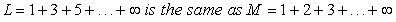Both series diverge to the same intuitive infinity though M series is the sum of natural numbers and has twice as many elements as in L series. This abandoning of diverging calculations and evoking the heuristic/ intuition called infinity qualifying both series as the same infinity, is the root of cause of our inability to make sense of the concerned mathematics. This will become clear in the subsequent sections, especially the section 4 on Cantor’s Diagonal Argument. A mathematical proof of “dissimilar cardinality” and the subsequent problems in mathematics is proven in the section 4 on Cantor’s Diagonal Argument.

Given any 2 finite numbers a and b, both greater than 0, their sum a + b > a, a + b > b is true. However, if b is infinite, regardless of what finite value a assumes, a + ∞ = ∞. The finite number a is swallowed up by the infinite. Infinite numbers were rejected as inconsistent. This was condemned by Georg Cantor as fallacious because it assumed infinite numbers to follow same arithmetic characteristic as finite numbers 5.

The axiom of infinity which is one of the axioms in Zermelo – Fraenkel Set Theory is the formal statement of the above inconsistency or incoherence of infinity in mathematics and the subsequent intuition,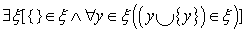Where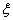is the infinite set, {} a null set and y is the member set of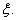The axiom of extension states that 2 sets are equal if and only if they have same elements 6. The formal statement of axiom of infinity above contradicts axiom of extension. This will be discussed extensively in this article.

Two themes are central to the article – 1) a priori intuition (understanding) which develops after abandoning the recursive expansion and contraction process and 2) assuming same cardinality of various “infinities” which will be discussed and proved later in the article.

Our cognitive processes, of course, will produce the understanding of infinity but if the cardinalities of all infinities are considered to be the same (just a flat infinity), mathematics will produce incoherent, inconsistent results and mathematics may not be able to yield a satisfactory treatment of infinity. The further inference from this article is that it is possible that this understanding of infinity is incorrectly applied to the physical reality.

I believe true progress of science is through combining of seemingly unrelated concepts and challenging our intuitive heuristics and then test it empirically. This article challenges the intuition of infinity and calls it, possibly, only a product of mind and attempts to deny, plausibly, the existence of physical infinity in the universe.

### 2. Sum of Infinite Sets

Ramanujan Summation: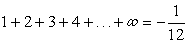The above derivation of Ramanujan Summation explained by 7 shows that sum of positive natural numbers is a negative fraction! Application wise, Ramanujan Series has been used in “Bosonic String Theory” and in the solution to the phenomenon called “Casimir Effect”.

To prove the above summation, we start by proving 2 more series,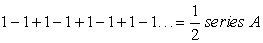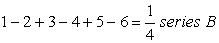For series A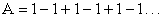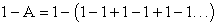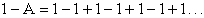which is equal to A again

So,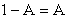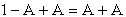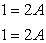So,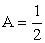this series is called the Grandi’s Series

Let,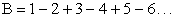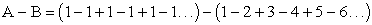Shuffling the terms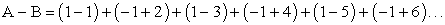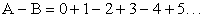So,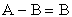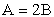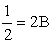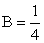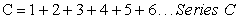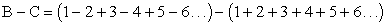Rearranging the terms,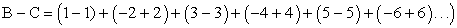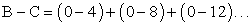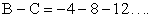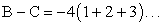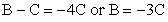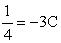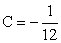Hence,Let us consider the series AGeneralizing,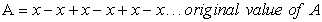(1)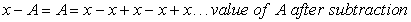(2)

Subtracting equation 1 from equation 2 (subtracting first element from equation 1 from first element in equation 2, maintaining the 1 to 1 correspondence of elements),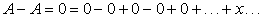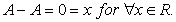This is the inconsistency of Grandi’s series and hence the derived Ramanujan’s Summation. The problem arising from assuming same cardinality of all kinds of “infinities” is discussed and proven in the section 4 – “Cantor’s Diagonal Argument”.

The explanation of why this is so is counter-intuitive but I will try to present the above mathematical argument in as verbal manner as possible. Because human mind can hold a finite object in the mind and expand it to an infinite size within the mind, in the series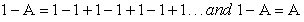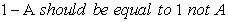But,andare considered equal in the derivation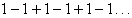and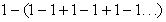appear same because of our mind’s ability of expand the finite to the size of infinite and reduce the infinite to the size of finite. The value heuristically remains the same only if the number of terms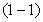are infinite. If it is possible to subtract the series A from 1, it is certainly possible to subtract the equation 1 from equation 2.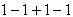and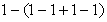are not the same. The infinity is a shortcoming or advantage of our intuitive understanding to stop the process of continuous expansion of a finite concept to the size of infinity and again reducing the expanded concept to a finite size and again expanding the finite concept to the size of infinity, ad infinitum, within the default network of the brain.

The value of Grandi’s series should be 0 not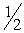for large or infinite number of termsif cardinality is considered correctly.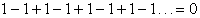### 3. Size of the Universe

If the universe is infinite at current time t, then it was infinite t-1, t-2, t-3.....hours ago. So, it was always infinite. This is the problem with the infinity as explained throughout this paper. Our mind can reduce the size of infinite concept to a finite size within the imagination network and again expand that finite size to infinity and repeat this procedure continuously until the mind abandons the process and declares the concept as infinite.

The Sun is probably not burning for infinite time and certainly the energy dissipated and scattered in the universe by the sun does not accumulate or converge into a sun again by second law of thermodynamics (increase in entropy), unless Steady State Theory (SST) or its variant Quasi-Steady State Hypothesis (QSS) by Hoyle Fred, Burbidge Geoffrey and Narlikar Jayant in 1993 8 which posit continuous creation of matter in the universe, is true. Even if the sun was created from a supernova of another star, that star must have had an origin. Going all the way back, we know our universe originated 13.8 billion years ago. So, it is more probable that we live in a finite physical world. There should be no such thing as infinite or infinitesimal in the physical world.

At present most cosmologists are pretty certain that the universe is finite, expanding from a point easily calculated from well-established measurements but the philosophical choice of whether the universe is finite or infinite remains unsolved 9. This will remain a conundrum, until we are able to travel to the edge of the universe. In his first assumption, Borchardt states that “mathematics really cannot yield a satisfactory treatment of infinity, so an assumption of finity comes natural. The problem is that, if one assumes finity at the beginning, one will end up with finity at the end. The argument becomes circular no matter which assumption one uses”. I think, we are confusing the logic with empiricism. The heuristic of infinity, a logical intuition, is brain’s way to stop the never-ending process of expansion and contraction of a concept in mind as discussed earlier. All infinities are abandoned as same infinities, even the ∞ +1 is abandoned as the same ∞. Just because the brain short-circuits the recurring concept to infinity does not mean the universe has to be infinite.

The third assumption, postulates that Big Bang Theory (BBT) is a blatant violation of conservation of energy and momentum 9. The conservation of energy and momentum is the law of our universe, a law which was probably created only after or when the universe was created. Let us define as absence of mass, energy, time, space and laws of physics. This “nothing” defined counter-intuitively, is where, plausibly, the causality can break down generating “effects” which are not spatially or temporally connected with each other (Quantum fluctuations in the “nothing” due to uncertainty is popular theory). “Effects” can occur without cause randomly in “nothing”. Example if a universe was created from “nothing” randomly after break down of causality at time t0 and another universe was created similarly at time t1 time will automatically emerge in “nothing” because the 2 “events”, if I may call it, are distinct events. But, by definition, “nothing” lacks the concept of time. So, the 2 universes in the multiverse are not temporally related to each other. There is no relation describing one as happening before other or vice versa. The same rationale can be applied to space. Distance will automatically emerge if 2 universes are considered to be located at 2 places in “nothing”. So the universes in “nothing” are not spatially related either. This preserves the definition of nothing. The instability of “nothing” due to uncertainty presumes the existence of “Heisenberg’s Uncertainty Principle” in “nothing”. If that is true, how should we explain the origin of “quantum theory” which accordingly pre-exists the universe, ad infinitum? Did quantum theory emerge from something else or it is the most fundamental entity in the “nothing”? 10.

• Figure 2. Incorrect representation of distance in a multiverse
• Figure 3. Incorrect representation of time in a multiverse

Also, referring to Figure 3, let the 2 universes emerge at 2 distinct “times”. Then what follows is, when universe 2 is created, the universe 1 may have been already created, is in the process of creation, or yet to be created, given that the temporal relationship between universes in the “nothing” does not exist. Similarly, in relation to universe 1, the universe 2 already has, is being or will exist in the future at a given instance. To put it another way, time is a concept local a universe. Distance and time cannot be seen in ways we see it inside a local universe. These space and time concepts should emerge after an individual universe is created 10. The multiverse can have infinite number and variety of universes 11 but the concept of time should only come into existence after an universe, such as ours is created. The multiverse cannot be an infinite universes which have been and are going to be produced for infinite time. In “nothing”, the parallel universes will exists before one another or together at the same time. There is no time to discriminate the existence of one universe before another or the many universes are temporally unrelated (“only in nothing”) in the multiverse, thinking in a counter-intuitive manner. This article supports the characteristics of BBU listed in the Table 1 in 9 as it challenges the concept of infinity as probably just a brain’s logical error which probably does not exist empirically.

Imagining “something” in the mind necessitates “something” to be “called” into the mind which totally contradicts with the concept of “nothing” which is absence of “something”. You cannot evoke “nothing” in the mind without creating “something” representing “nothing”. Hence it is impossible to imagine “nothing” in the mind. This makes the concept of “nothing” very counter intuitive and is that leads to its denial along with the theories tied to it like BBT, as the origin of our universe.

Apart from Hubble's law which is considered as the first observational basis for the expansion of the universe and abundance of light elements, the Cosmic Microwave Background Radiations (CMBR) which are the remnant electromagnetic radiations of the early universe is important evidence of BBT.

Today’s systems philosophy, such as BBT tends to isolate a portion of the universe by pointedly ignoring its environment. All of the causes for the effects observed within a particular system are considered to have originated within the system itself. The theory assumes that the entire universe, like other systems, is finite, had an origin, and will have an end (Borchardt, Glenn, 2007). The “portion of universe ignoring its environment” the author is refering to is the universe and “nothing” “outside” it. This is the classical intuitive/ heuristic concept of contracting and expanding of universe in the mind and infinitely questioning about the “outside” of the universe and concluding the process to assert that universe is infinite. The “univironmental determinism” applies to a microcosm and macrocosm within the universe but universe as a whole does not need to have the “outside” or macrocosm. It does not necessarily apply to universe as a whole. The law of physics and reason came after the universe was created and hence will work for systems within the universe and not to the macrocosm outside the universe, as conceptualized in 12.

In 1963 Arno Penzias and Robert Wilson, studying the sky's microwave “noise” for Bell Telephone Laboratories, realized that they had detected microwaves coming from all around the sky, a universal background radiation. Also, Robert Dicke, a physicist nearby at Princeton University, learned of the measurement and in 1965 correctly interpreted it as radiation of about 3 degrees Kelvin, left over from the big bang. According to the SST, the CMBR can possibly be the ancient light from stars scattered by galactic dust. However the SST does not explain the uniformity of CMBR in all directions and non-polarization.

By WMAP data it is known (as of 2013) that the universe is flat with only a 0.4% margin of error which suggests that our universe is infinite in extent. However, as our universe has a finite age, we can only observe a finite volume of our universe 13. The only thing that can be necessarily concluded is that the universe is much larger than the volume we can directly observe.

It is possible that the universe did not emerge from singularity but “infinite universe” was created from big bang but does the concept of “infinite” universe emerge from the mind’s a priori concept of infinity?

“The discovery that the universe is not static, but rather expanding, has profound philosophical ….significance, because it suggested that our universe had a beginning…. The Cosmic Microwave Background Radiation (CMBR) is nothing less than the afterglow of the Big Bang. It provides another piece of direct evidence, in case any is needed, that the Big Bang really happened, because it allows us to look back directly and detect the nature of the very young, hot universe from which all the structures we see today later emerged” 14.

### 4. Uncountability of Infinite Sets

Cantor’s Diagonal Argument:

Though in 1874, Cantor published his first proof of the existence of transcendental numbers 15, 16 discussed in the article 17, this paper will analyse the Cantor’s Diagonal argument 18 and reference (“Cantor’s Diagonal Argument” 34). The paper will first discuss Cantor’s Diagonal argument briefly and then provide the mathematical proof of why uncountability/ transcendental numbers exist only in mind, the problems with method used to prove uncountability/ transcendental numbers and provide some reinforcement to the claim of this paper that “infinity” probably does not exist in the physical world.

T is a set of infinite sequences of binary digits. s1, s2, s3,….sn…is any enumeration of elements from T. For example,Now, a new sequence is created by taking the opposite of a different element from each of the above set starting from 1….7…… So, the new diagonal set will be (1, 0, 1, 1, 1, 0, 1…) which is obviously different from any of the sets lists above from s1 to s7…..as we took the opposites. We proof starts with the hypothesis that T is countable and then generates a sequence which is not in the enumeration but as any s is an element of T, the contradiction falsifies the hypothesis. By creating an injection (mapping function), it is shown that as T is uncountable, R (real numbers) is uncountable as well.

If these sequences listed above were finite number of finite sequences with the same 7 elements then,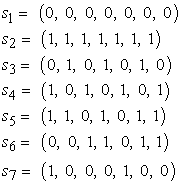The total number of sequences possible with 7 binary numbers is 27 = 128 while the number of sequences listed above is only 7 (s1 to s7 to form a diagonal). Just to clarify, the above statement has nothing to do with Cantor’s theorem of cardinality of power set of a set, both finite and infinite. Here, 27 = 128 is not the number of power sets but total number of sequences possible but varying the bits 0 and 1. Because every element in set s can take up either 0 or 1 value, the total number of sequences possible is 2n. Clearly, there will exist sequences which are not listed above. Generating a new diagonal sequence similar to the one generated above for infinite sequences we get (1, 0, 1, 1, 1, 0, 1) which is, of course, not listed above. So, regardless of whether the sequences are finite or infinite, there will be sequences which are not listed in the list of sequences (as above). List of 128 sequences each containing 128 elements will also have new sequences which are not part of sequences listed because 2128 > 128. To obtain a diagonal, the number of elements in each sequence should be equal to number of sequences. This clearly is not possible because for n elements in each sequence there are 2n total sequences. But both, the number of elements in each sequence and total numbers of sequences are same if both are considered to be infinite. So, the diagonal argument seems to work for infinite elements in each sequence and infinite such sequences.

Let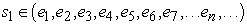where, e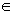{0, 1}.

Hence the cardinality (c) of e is 2.

Let,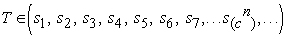To get a diagonal the matrix, cardinality of s should be equal to T should have,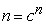(3)

But ξ (c, n): cn > n, where n is a positive integer and c ≥ 2.

When c =2 and n =1, ξ (2, 1) is true as 21 > 1.

Similarly, ξ (2, 2) is true as 22 > 2.

By induction,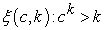multiplying both sides by c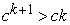but ck ≥ 2k so,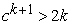since (2k = k + k ≥k + 1), ck+1 > k +1.

Hence,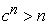But, by equation 1, it is required thatbut if T and s considered are infinite, n = cn = ∞ (an intuition which qualifies and quantifies n = cn but does not appear to be true.)

The cognitive processes can certainly conceive of uncountability of T and R. The proof does not say that the sets T or R which has a bijective mapping with is not uncountable. It only explains the shortcomings of assuming the same cardinality to all infinities.

Infinity appears to be possibly an intuition/ heuristic only in the human mind allowing us to obtain the same number of ∞ elements in each sequence (n) and total number of sequences (cn) and hence prove the uncountability of T. The cardinalities of elements in a sequence and total number of such sequences become equal if both the sets are considered infinite. It is the abandoning of recursive expansion and contraction process and the subsequent intuition of infinity that allows us to prove the uncountability of T or R.

Also, according to Cantor, it is possible for infinite sets to have different cardinalities, and in particular the cardinality of the set of real numbers is greater than the cardinality of the set of natural numbers. It is also possible for a proper subset of an infinite set to have the same cardinality as the original set which is not possible with proper subsets of finite sets (“Cardinal Number” 35). The root cause of incoherence in the mathematics of infinity is that the intuition of infinite is same for all infinities.

The proof above does not attempt deny the existence of the concepts of infinity and infinitesimal in our cognitive processes. But, if the cardinalities of all infinities are assumed to be same, as our intuition of infinity suggests, the subsequent mathematics will lead to inconsistent results. The purpose of this article is also to show that infinity may be only a cognitive concept and it is incorrectly applied to the physical reality (though infinite abstract cyclical processes are possible in the mind).

### 5. Quantum Field Theory & Mind Functioning & Other Perspectives

In the interesting article 19, “The emergence of the mind as a quantum field phenomenon”, it is proposed that quantum fluctuations in the subatomic fields within the quantum vacuum space of the neural networks results in the generation of a signal or action potential between the neurons. The mind is the result of interaction of subatomic quantum fields within the neural cells which computes and supports various brain processes. The intensity of computations is different from cell to cell. Some structures like brain or heart have higher intensity while the skin has less intensity. Also, the memory is created within these signals or interactions and is embedded as sensory, short term or long term memory which becomes part of the mind. Creation of thoughts is a consequence of quantum fluctuations caused by these quantum field interactions in the quantum vacuum. These event which happen in our body, do not happen in the domain of consciousness because these events only become conscious when organs malfunction. Mind is a subtle non-physical system which has evolved as an outcome of certain organizations and patterns of quantum interactions in the associated physical system. Can our understanding which surpasses machine understanding 20 be due to the quantum processes (interactions) in the brain (and other structures)? Is the understanding of infinity a result of fluctuations arising from interactions of quantum fields? What is the right reduction, biological/neural structures or physics? Is the qualia experienced by the quantum fluctuations arising from quantum interactions of subatomic fields?

The implicit mental imagery, like imagining a cube in the mind’s eye, is perceived differently than perception of an actual physical cube with the physical eyes. The implicit imagery lacks localization information which is one of the parameters in a sophisticated information processing device like brain, the others being qualia nature, discrimination and externality, to generated rich, vivid image like the one seen while observing an actual cube. “The parameters of Qualia and Neural Code” 21 and the continuation “Quantum Interpretation (Physics) – Information Field”, 10 suggests the plausibility of collapse of the wave function only in the information. The information field only populates or attributes those information values to the information field which are available. The implicit imagery of a cube experienced in the mind’s eye is different from the actual perceptual experience of a physical cube. The externality parameter which tells if the experience is felt outside (like the visual perceptual experience is felt outside the body or pain experience which is felt at the location of injury) is zero during implicit imagery in the mind’s eye. The discrimination is not as discriminated as the actual perceptual experience but the qualia nature (visual in the case of a cube) is present. The information field acquires different values of information in both the cases.

In dissipative quantum model of the brain, the brain–environment entanglement is a specific dynamical feature of the brain functional activity 22. Consciousness finds its roots and resides in the persisting “dialog” between system and environment. as a consequence of Spontaneously Broken Symmetry, long-range correlations are dynamically produced in the system . The system’s ground or vacuum state is then a coherent condensate of the Nambu–Goldstone (NG) quanta, associated with such correlation waves. These NG quanta are massless so that the associated correlation waves may span the whole system volume, taken to be very large (infinite in the QFT limit). The external input received by the brain triggers the SBS and the consequent dynamical generation of the long-range correlations, whose NG quanta coherently condense in the vacuum. Memory is the printed ordered pattern generated in this way.

The APOS (Actions, Processes, Objects, Schema) theory explains the concept of our understanding of infinity by mechanisms such as interiorization, encapsulation and thematization. In an example of finding the behaviour of quadratic equation in a given interval, the transformation of calculating the functional values over the interval is first conceived as an action. Reflecting upon the relationship between function values leads to interiorization of action into a mental structure called process. On awareness of the process as totality, the process becomes encapsulated into a cognitive object. The objects, then also need to be linked and organized into a coherent framework called schema 23.

In order to discuss infinity one must have a concept (or concepts) of infinity. Without it, it would not be possible to say anything about infinity, even that it does not exist. Without acquirement of a concept we would not be able to tell if it is, e.g. self-contradictory 24. According to intuitionist doctrine the mathematical universe is human-made. According to Brouwer even the universe does not have a-priori existence. For intuitionists, mental processes is the only source of construction of abstract objects. The existence of an abstract object is same as the possibility of its construction by a human mind. Mathematical statement corresponds to a mental construction, and a mathematician can assert the truth of a statement only by verifying the validity of that construction by intuition. The activity of the mind has its origin in the perception of the movement of time. Time – in the Kantian sense – is the only an a priori notion. Awareness of time is indispensable in the process of mathematical cognition, or equivalently in the process of creation. The mental construction of one natural number after the other needs an awareness of time within us. Mathematics is the intrinsically subjective activity of an individual mathematician’s intuition. The communication between mathematicians only serves as a means to create the same mental process in different minds. Brouwer rejected the use of formal systems entirely saying that they are irrelevant for mathematical reasoning. The role of language as a tool of mathematical cognition – indispensable in the case of the formalists – was questioned. The abstract mental constructions are independent from language. Mathematics is a languageless creation of the mind 25 and 26. This understanding is very close to the theory presented in this article.

### 6. Infinity in “nothing”

The popular theory of creation of our universe through (BBT) is the uncertainty or instability of “nothing” which punches out universes like our own from “nothing”. However uncertainty principle is a law of physics and we may very well question the origins of this uncertainty. This “nothing” with the uncertainty is shown in Figure 4 below as Nc3. Ideally, such Nc3 should originate from a “nothing” without any laws of physics (N).

• Figure 4. Depicts infinity in the “nothing”

However, if no laws govern “nothing”, it should be able to create anything and nothing. As soon as there are laws in “nothing”, the origin of those laws need to be explained. If there are no laws in “nothing”, it would mean that there are infinite possibilities being created from such a N. This is possible in N because it is not a physical universe. The causality, that cause precedes the effect is only a law of our universe but in “nothing” the causes can occur “after”, if I can use the word, or “simultaneously with” the effect. One universe would be where matter spontaneously assembles into a configuration of tree without natural selection. The space time axes are shown in the Figure 4 to depict that the various ‘nothings” are not spatially or temporally related. Ideally, rather than stacked upon each other, they should be on each other. There is no z axis. If N can give birth to infinite possibilities, one of the 2 important possibilities is that it gives birth to an Nc1 which is the cause of N. So, the N creates a cause of itself, the cause which is created by causes other than N, such as Nc2 which has a cause Nc4ad infinitum. The other important possibility is where N gives birth to Nc3 which can cause the N forming the loop. The 2 possibilities are not flowing in space or time. So, the creation of N is an on-going infinite process which means that “nothing” is in a process of creation. The above discussion is counter-intuitive and the theory of “nothing” should be counter-intuitive because intuition evolved to understand our physical universe not the “nothing”.

### 7. Other Infinite Intuitions

Example 1:

The first of the above 3 positions, Figure 5, is a draw even though black is 2 rooks and a piece up. The rooks and the bishop cannot cross the closed pawn chain and can therefore never mate the white king. Roger Penrose made a point using the above chess position that human understanding surpasses the “mere” calculations of chess engine 20. However, I want to add to this by saying that human brain can also abandon the infinite calculations by generating an intuitive understanding that the given process is never ending. The understanding in the above chess position is similar to the understanding of abandoning the infinite process just because it never ends. In the above chess position, the intuitive understanding is that the black pieces cannot cross the closed pawn chain. In this case, the intuition is correct however it is possible that we may abandon the calculations and generate an intuition which is false.

The second position in Figure 6 is also a draw as the fortress created by the coordinated activity of the Knight and the Bishop guards the squares a3, b3, c3, c2 and c1 and black king cannot enter the fortress. The white king cannot be put into a zugzwang with a stay move either.

In both the above positions, the intuitive short-cut turns out to be right. However, the intuitive heuristic does not necessarily have to be right which is explained with the third position.

In the third position in Figure 7, it is black to play and the Grandmaster playing black pieces resigned the game because his knight is trapped on h3 and will be eventually taken by the white king. However, if the black king walks to the a8 square, it is a draw! The position is available in the table bases as a draw. In the third position the intuition is that the knight on h3 is trapped by the white bishop controlling g1, f2, f4, g5 squares available for the knight. Also, the white king can approach the knight on h3 through g4 and take the knight. However, there is another understanding which is difficult to see that the black king on a8, with the given pawn structure on b7, b6 and a dark squared bishop who cannot take the b7 pawn will lead to a draw. This example is about abandoning the calculation process too early to generate a wrong heuristic or applying incorrect heuristic (like in the third position) and generating possibly wrong conclusions (like in understanding the size of universe).

Example 2:

In quantum mechanics, we know that a particle can exist at 2 places at the same time. This defies logic! Logic evolved to represent the universe as accurately as possible. But, we did not evolve to understand quantum mechanics, we evolved to survive (survival of genes) and reproduce (which is just another way to survive). We never worked on the quantum scale to evolve logical understanding of quantum mechanics. This is another erroneous, a priori, understanding of reality however infinity is a mind’s intuitive error not a logical one.

Example 3:

The biases of intuition, which he calls as system 1, have been comprehensively discussed in the seminal book “Thinking Fast and Slow” by 27. Abandoning the recursive contraction and expansion process while contemplating the never ending process and then the declaration of the intuitive answer is also possibly a bias of intuition. The non-stopping nature of the computation cannot be established by any set of clear cut rules ( 20, pg 72). The book uses Godel’s Theorem to show that human understanding cannot be an algorithmic activity. Though the book, to the best of my knowledge, does not discuss the intuition of infinity and in particular the “size infinity”, the experimental methods suggested in section 10 should be used to understand the neurological basis of size infinity.

### 8. Infinity in Limits, Calculus, Irrationals and Series

The derivative is the instantaneous rate of change of a function with respect to one of its variables. The geometric definition of derivative as tangents is as below,

• Figure 8. Geometric definition of a derivative as tangent

P (x, f(x)) and Q (x+h, f(x+h)) are 2 points close to each other on the curve y = f(x). PM and QM are perpendiculars from P and Q on x axis and PL as perpendicular from P on QN. The chord PQ is produced to meet x axis at R and angle QPL = angle QRN = Φ. In QLP,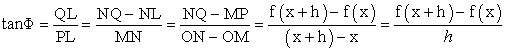Taking limits h →0 on both sides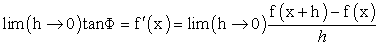which is the first principle.

Example,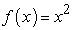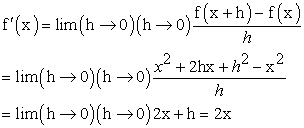The derivative is not 2x. It is 2x + h. Or 2x + 0.0000000000……1

With the very large number of zeros, the derivative approximates to 2x.

We abandon the process of putting zeroes after zeroes for large number of times and declare the answer as 2x. This works in calculus to get an approximate answer. Bishop George Berkeleyhad objected in the favour of the calculation of derivative as mathematically legitimate if h is considered as a true number as against eliminating it when needed 2. Newton gave the definition of derivative far off the mark not to satisfy the objection above. Yet, as the infinitesimal value approaches a number, the approximate solutions in calculus work.

Even though the number of zeroes approaches infinity, in general, a convergent series will have a mathematical treatment, as the sum converges to a number.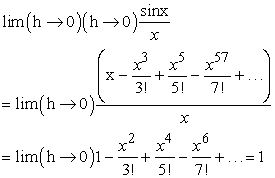Geometric series is another example of convergent series with applications in engineering, physics, mathematics etc. Empirically, an electron is regarded, so far, as a fundamental particle which exhibits both wave and particle nature. Any further, intuitive subdivision, which may work intuitively, is not known to be physically possible. The infinitesimal is 1/ ∞ follows the same intuition as infinity ∞.

The irrational number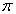is non-terminating and non-recurring number with infinite digits following the decimal point. There is a smaller infinitesimal increase in the value ofwith every digit. This converges to 3.14 but never becomes 3.15. Just like in convergent series, the digits in an irrational number approach or tend towards a number hence meaningful mathematics is possible even though infinite number of digits is involved.

Galileo presented his paradox in the example of numbers and their squares, called Galileo Paradox. Every number is a square (root) of some number and there is a bijective relationship with infinite such bijective pairs. But as lot of numbers are not squares there are more numbers than squares (roots). Galileo resolved the contraction by stating that the comparison is only relevant to finite things and not to infinite 28. The intuition collapses any recurring concept to infinity, which is one of the two recurring themes of this paper, the other being, assuming same cardinality for all infinities!

9.2. Hibert’s Paradox of the Grand Hotel

The Hilbert’s Paradox of Grand Hotel is briefly discussed in 29. The Grand hotel has infinite rooms all of which are occupied. When a new guest arrives, the guest from room 1 is moved to room 2, from room 2 to room 3 and the guest from nth room is moved to n+1th room. The hotel with n rooms is assumed to be infinitely large and the same hotel with n+ 1 rooms is also infinitely large, where infinity corresponds to the same concept even though a room is added to the hotel. The recursive process is abandoned once again to the same intuitive concept of infinity. ∞ and ∞+1 do not correspond to 2 things but the same infinity. The paradox will occur if the cardinality of all infinities is assumed to be same. Also, this paradox is another abstract concept of infinite rooms in the mind and not possible in the physical world.

Escher’s square limits, circle limits, Path of Life, Stairs, Drawing hands creatively depict infinity and paradoxes. M.C. Escher’s depictions of infinity fall into three main categories: limits, endless cycles, and regular divisions of a plane 3. Max Bill created a sculpture of a Möbius strip, which he called Endless Ribbon 3. Sir David Brewster, a Scottish physicist, invented the kaleidoscope to depict repeated designs 3. Douglas Hofstadter 30 gives an example of strange loop in music from Bach’s Musical Offering.

### 10. Proposed Empirical Tests (Theoretical Illustration)

The Default Network comprises of medial prefrontal cortex, posterior cingulate cortex (PCC), inferior parietal lobule (IPL), lateral temporal cortex (LTC), parahippocampal cortex (PHC) and hippocampal formation (HF) connected with different region to region correlation is responsible for internally focused tasks 31. The Default Network works closely with the Central Executive Network and Salience Network in the holistic imagination process. Spatial navigation and processing of concepts like infinity including the subsequent heuristic/ intuition should involve default network. The visualization of infinity should start with visualization of an infinite concept in the default network but within imagination, this concept which stretches across the imagination or “mental workspace” can be shrunk to a smaller size in a background (called “outside” earlier in this article). This shrunken concept in the mind with its background together becomes new infinity which can be shrunk again. This process continues indefinitely until a heuristic (intuition evolved for reaching conclusion in a never ending process) stops the process. The heuristic can stop the process after any number of iterations which is why all infinities are same. ∞ is same as ∞ + n.

• Figure 9. (e1, e2, e3, e4) represent the 4 ensembles of neurons depicting expansion and contraction process. This is the illustrative neural representation of Figure 1 in section 1 with each 4 figures in Figure 1 corresponding to the above 4 figures in Figure 9 respectively

The neurobiological substrate of the imagination process which combines and modifies stored perceptual information (explicit imagery) and concepts leading to creation of novel mental objects could be found in a hierarchical model of the brain characterized by a multiplicity of functional modules (interaction dominant dynamics system) that can be assembled according to different spatial and temporal scales. It is surmised that a possible mechanism for the emergence of imagination could be represented by modulatory mechanisms controlling the openness of modifiers along the communication channels within and between functional modules leading to their dynamically reassembling governed by spatial location and order of activation into imagination 32. Imagination is not just organization, identification and interpretation of imagery but a constructive process creating new view of the world, speculation and simulations.

The successive expanding and contracting of an infinite size concept, such as universe, is shown in Figure 9. The concept in its expanded (infinite) form is shown in e1 (by red neurons). The imagination or default network can reduce this to a smaller size as shown in e2 (shown by red neurons), where it can imagine and question what is beyond the small concept. The whole network e2 is expanded again to the full size or infinity in e3 (shown by red neurons) and e3 can be reduced to a small size (shown by red neuron), ad infinitum. This process will continue when we imagine, for example, universe until the heuristic/ intuition abandons the process and concludes infinity. Thus, this recurrent expansion and contraction of concepts in the mind will be followed by a firing of finite neural network representing infinity.

If the imagination network is not able to switch from e1 to e2 or e2 to e3 or e3 to e4, the mind will not be able to imagine infinity.

Though, the brain scan can be performed to see these oscillations, it still can just be a trait evolved in the brain to represent external reality. These oscillations are not the gamma, beta, alpha waves found in the EEG. The oscillations in the context of this article are the brain’s ability to perform iterations from e1 to e2 and e2 to e3 and e3 to e4 until the brain stops these iterations and declares infinity. So, the scans would still not prove that size infinity exists only in the mind. The real empiricism is to perceive the edge of the universe in order to know if “size infinity” exists in the physical world. Also, the scans need capture the “size infinity” neurological processes or the neural network of the heuristic not the cyclically infinite visualization processes such as that of an abstract sine wave. Also the neural network responsible for defaulting to the infinity heuristic should be located (left lateralized frontal-temporal conceptual/language system processes familiar, conceptually coherent material, corresponding to the heuristic system 33), which should be the same network, both in case of an abstract sine wave or contemplation of infinite universe.

### 11. Conclusion

When you shrink an infinite concept to the size of a peanut in your mind, there is always something outside the peanut in the mind. The whole thing, the peanut and the outside of the peanut can be shrunk down again to a size of another peanut, ad infinitum until your brain abandons this process and creates an understanding of infinity. There is a persistent, compelling intuition that infinity exist, but this paper has presented that this infinity probably exists only in the mind. At the edge of our universe, peeping outside, one will see “nothing”, in spite of the stubborn intuitive feeling that there should be something beyond the edge.

In this research paper, the on-going debate of infinite vs. finite universe with supporting arguments is presented. The SST is has lost its popularity as discussed in section 3 and BBT with a plausible origin of universe from “nothing” has gained ground. Without the laws of physics, in nothing, it is plausible, though counter intuitive that our universe originated from nothing. Infinity probably is just a creation of the mind. Or in other words, it can be the flexibility of an evolved mind that creates a persistent, compelling concept of infinity.

The Ramanujan’s Summation and Cantor’s Diagonal Argument are presented in the new light. Is the intuition of qualifying any never ending process as infinity giving rise to erroneous mathematics? However, it is also shown that limits, calculus and convergent series converge to a finite approximate number without any problems.

It is shown that mathematics remains meaningful and coherent when the infinite digits convergent to some number and does not expand unbounded and indefinitely, in the section 8 on limits, calculus, irrationals and series.

There are many other similar intuitions and logical fallacies, some which are presented in section 7. Infinity is proposed as a possible intuitive/ heuristic error in this article. This intuitive error probably manifests in all the popular paradoxes discussed in section 10 as well.

Of course, the theory presented here is not empirically supported today but some brain scans are suggested in section 10 to support the theory. Though the brain scan cannot falsify the existence of infinite or infinitesimal physical reality as it is possible that the neural networks evolved to mirror physical reality, it reinforces the idea that, possibly, infinity exists in the mind.

### References

  Hilbert, David. (1926). ‘Uber das Unendliche’, Mathematische Annalen. 95, 161-190. In article View Article  Donald, Allen. (2003). The History of Infinity. Texas A & M Mathematics. Retrieved 2019-11-15. In article  Batchelor, John H. (2015).The History and Evolution of the Concept of Infinity. 10.13140/RG.2.1.4350.1284. In article  Secretary. (2006). Mathematics, Textbook of Class 9th. National Council of Educational Research and Training, 2006. In article  Dauben, Joseph W. (1979). Georg Cantor: His Mathematics and Philosophy of the Infinite. Princeton University Press. In article  Halmos, Paul R. (1960). Naïve Set Theory. Litton Educational Publishing, Inc. In article  Dodds, Mark. (2018). The Ramanujan Summation: 1+2+3+4…+∞ = -1/12?. Ramanujan Essays. Cantor’s Paradise, A weekly Medium Publication. In article  Hoyle, Fred. Burbidge, Geoffrey. Narlikar, Jayant V. (1993). “A quasi-steady state cosmological model with creation of matter”. The Astrophysical Journal. 410: 437-457. In article View Article  Borchardt, Glenn. (2015). Infinite Universe Theory. Conference: Proceedings of Natural Philosophy Alliance. Project: Scientific Philosophy. (http://scientificphilosophy.com/Downloads/IUT.pdf). 4. 20-23. In article  Manohar, Abhijit. (2021). “Quantum Interpretation (Physics) - Information Field.” International Journal of Physics, 2021, 9 (5), 222-233. In article  Kaku, Michio. (2006). Parallel Worlds. Knopf Doubleday Publishing Group. In article  Borchardt, Glenn. (2007). The Scientific Worldview and the demise of Cosmogony. Proceedings of the Natural Philosophy Alliance. 4. 16-19. In article  “Will the Universe expand forever?”. National Aeronautics and Space Administration (NASA). 24 January 2014. https://map.gsfc.nasa.gov/universe/uni_shape.html In article  Krauss, Lawrence. (2012). A Universe from Nothing, Why there is something rather than nothing. Free Press, A Division of Simon and Schuster, Inc. In article  Cantor, Georg. (1874). Uber eine Eigenschaft des Inbegriffes aller reellen algebraischen Zahlen. J. Reine Angew. Math. 77 (1874), 258-262. In article View Article  Cantor, Georg. (1883). Sur unepropriete du systeme de tous les nombres algebriques reels. Acta Math. 2 (1883), 305-310. In article View Article  Gray, Robert. (1994). Georg Cantor and Transcendental Numbers. American Mathematical Monthly, 101 (9): 819-832. In article View Article  Cantor, Georg. (1991). Uber eine elementare Frage der Mannigfaltigkeitslehre. Jahresbericht der Deutschen Mathematiker – Vereinigung 1 (1891), 75-78. In article  Pereira, Contzen, Reddy J Shashi Kiran. (2018). The Emergence of Mind as a Quantum Field Phenomenon. NeuroQuantology, Nov 2018. Volume 16, Issue 11, Page 68-78. In article View Article  Penrose, Roger. (1994). Shadows of the Mind: A Search for missing Science of Consciousness. Oxford University Press, 1994. In article  Manohar, Abhijit. 2021. Parameters of qualia and the neural code. International Journal of Scientific and Engineering Research. Volume 12, Issue 6, June-2021, ISSN 2229-5518 In article  Sabbadini, Shantena A, Vitiello, Giuseppe. (2019). Entanglement and Phase-Mediated Correlations in Quantum Field Theory. Application to Brain-Mind States. Applied. Sciences. 2019, 9, 3203. In article View Article  Weller, Kirk. Brown, Anne. Dubinsky, Ed. Mcdonald, Michael. Stenger, Cindy. (2004). Intimations of infinity. Notices of the American Mathematical Society. 51. In article  Frege, G. & Austin, J. (1980). The Foundations of Arithmetic: A Logico-Mathematical Enquiry into the Concept of Number, Northwestern University Press. In article  Trzęsicki, Kazimierz. (2015). How are concepts of infinity acquired?. Studies in Logic, Grammar and Rhetoric. 40 (53) 2015. In article View Article  Brouwer, L. E. J. (1981), Brouwer’s Cambridge Lectures on Intuitionism, Cambridge University Press, Cambridge. In article  Kahneman, Daniel. (2011). Thinking, Fast and Slow. Farrar, Straus and Giroux, 2011. In article  Galilei, Galileo. (1638). Dialogues concerning two new sciences. Transl. Crew and de Salvio. New York: Dover. pp. 31-33. In article  Gamow, George. (1947). One Two Three... Infinity: Facts and Speculations of Science. Viking Press, New York. In article  Hofstadter, Douglas R. (1979). Godel, Escher, Bach: an Eternal Golden Braid. Basic Books Inc., New york April 1979. In article  Buckner Randy, Andrews-Hanna Jessica, Schacter Daniel. (2008). The Brain's Default Network. Annals of the New York Academy of Sciences. 1124. 1-38. In article View Article  PubMed  Luigi F. Agnati, Diego Guidolin, L. Battistin, G. Pagnoni, K. Fuxe. (2013). The Neurobiology of Imagination: Possible Role of Interaction-Dominant Dynamics and Default Mode Network. Frontiers in Psychology. 2013; 4: 296. In article View Article  PubMed  Goel, Vinod. (2009). Cognitive Neuroscience of thinking. Handbook of Neuroscience for the Behavioral Sciences. In article View Article  “Cantor’s Diagonal Argument.” Wikipedia, Wikimedia Foundation, 9th of August 2021, https://en.wikipedia.org/wiki/Cantor%27s_diagonal_argument. In article  “Cardinal Number.” Wikipedia, Wikimedia Foundation, 25th of August 2021, https://en.wikipedia.org/wiki/Cardinal_number In articleThis work is licensed under a Creative Commons Attribution 4.0 International License. To view a copy of this license, visit http://creativecommons.org/licenses/by/4.0/